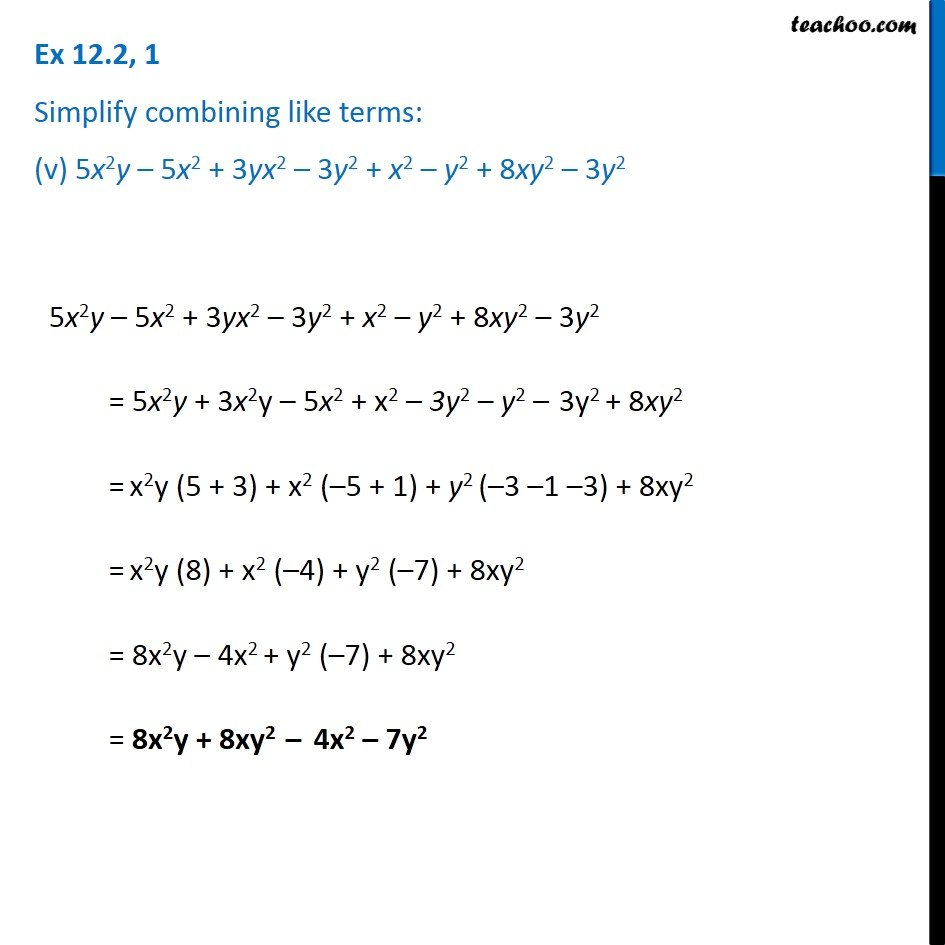Adding and Subtracting Like terms - Worksheet

Chapter 10 Class 7 Algebraic Expressions
Serial order wiseLearn in your speed, with individual attention - Teachoo Maths 1-on-1 Class

### Transcript

Question 1 Simplify combining like terms: (v) 5x2y – 5x2 + 3yx2 – 3y2 + x2 – y2 + 8xy2 – 3y2 5x2y – 5x2 + 3yx2 – 3y2 + x2 – y2 + 8xy2 – 3y2 = 5x2y + 3x2y – 5x2 + x2 – 3y2 – y2 – 3y2 + 8xy2 = x2y (5 + 3) + x2 (–5 + 1) + y2 (–3 –1 –3) + 8xy2 = x2y (8) + x2 (–4) + y2 (–7) + 8xy2 = 8x2y – 4x2 + y2 (–7) + 8xy2 = 8x2y + 8xy2 – 4x2 – 7y2